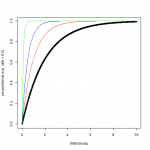Want to share your content on R-bloggers? click here if you have a blog, or here if you don't.On this set of exercises, we are going to explore some of the probability functions in R with practical applications. Basic probability knowledge is required.

Note: We are going to use random number functions and random process functions in R such as `runif`, a problem with these functions is that every time you run them you will obtain a different value. To make your results reproducible you can specify the value of the seed using `set.seed(‘any number’)` before calling a random function. (If you are not familiar with seeds, think of them as the tracking number of your random numbers). For this set of exercises we will use `set.seed(1)`, don’t forget to specify it before every random exercise.

Answers to the exercises are available here

If you obtained a different (correct) answer than those listed on the solutions page, please feel free to post your answer as a comment on that page.

Exercise 1

Generating random numbers.  Set your seed to 1 and generate 10 random numbers using `runif` and save it in an object called `random_numbers`.

Exercise 2

Using the function `ifelse` and the object `random_numbers` simulate coin tosses. Hint: If `random_numbers` is bigger than .5 then the result is head, otherwise is tail.

Another way of generating random coin tosses is by using the `rbinom` function. Set the seed again to 1 and simulate with this function 10 coin tosses. Note: The value you will obtain is the total number of heads of those 10 coin tosses.

Exercise 3

Using the function `rbinom` to generate 10 unfair coin tosses with probability success of 0.3. Set the seed to 1.

Learn more about probability functions in the online course Statistics with R – Advanced Level. In this course you will learn how to

• work with different binomial and logistic regression techniques,
• know how to compare regression models and choose the right fit,
• and much more.

Exercise 4

We can simulate rolling a die in R with `runif`. Save in an object called `die_roll` 1 random number with `min = 1` and `max = 6`. This mean that we will generate a random number between 1 and 6.

Apply the function `ceiling` to `die_roll`. Don’t forget to set the seed to 1 before calling `runif`.

Exercise 5

Simulate normal distribution values. Imagine a population in which the average height is 1.70 m with an standard deviation of 0.1, using `rnorm` simulate the height of 100 people and save it in an object called `heights`.

To get an idea of the values of heights applying the function `summary`to it.

Exercise 6

a) What’s the probability that a person will be smaller than 1.90? Use `pnorm`
b) What’s the probability that a person will be taller than 1.60? Use `pnorm`

Exercise 7

The waiting time (in minutes) at a doctor’s clinic follows an exponential distribution with a rate parameter of 1/50. Use the function `rexp` to simulate the waiting time of 30 people at the doctor’s office.

Exercise 8

What’s the probability that a person will wait less than 10 minutes? Use `pexp`

Exercise 9

What’s the waiting time average?

Exercise 10

Let’s assume that patients with a waiting time bigger than 60 minutes leave. Out of 30 patients that arrive to the clinic how many are expected to leave? Use `qexp`